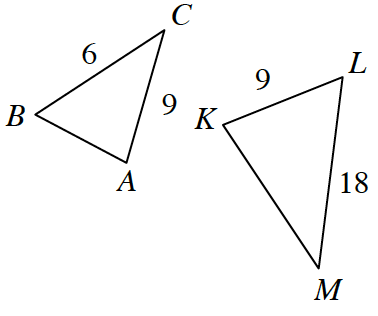### Home > CCG > Chapter 8 > Lesson 8.3.3 > Problem8-129

8-129.

Multiple Choice: The diagram at right is not drawn to scale. If $\Delta ABC \sim \Delta KLM$, find $KM$.

1. $6$

1. $12$

1. $15$

1. $21$

1. None of theseThe ratios of corresponding sides are equal if two triangles are similar.

The ratio of corresponding sides is equal to $3$.